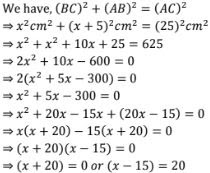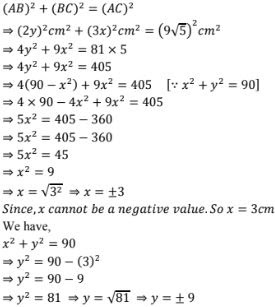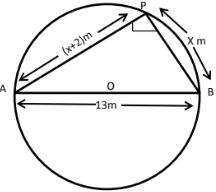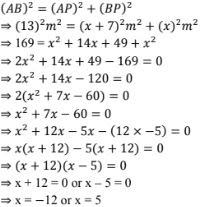# RD Sharma Solutions Chapter 8 Quadratic Equations Exercise 8.10 Class 10 MathsChapter Name RD Sharma Chapter 8 Quadratic Equations Book Name RD Sharma Mathematics for Class 10 Other Exercises Exercise 8.1Exercise 8.2Exercise 8.3Exercise 8.4Exercise 8.5Exercise 8.6Exercise 8.7Exercise 8.8Exercise 8.9Exercise 8.11Exercise 8.12Exercise 8.13 Related Study NCERT Solutions for Class 10 Maths

### Exercise 8.10 Solutions

1. The hypotenuse of a right triangle is 25 cm. The difference between the lengths of the other two sides of the triangle is 5 cm. Find the lengths of these sides.

Solution

Let the length of the shortest side be x cm
Given that the length of the largest side is 5 cm more than that of smaller side
⇒ longest side = (x + 5)cm
And also, given that
Hypotenuse = 25 cm
So, let us consider a right angled triangle ABC right angled at B
We have, hypotenuse (AC) = 25 cm
BC = x cm and AB = (x + 5) cm
Since, ABC is a right angled triangle2. The hypotenuse of a right triangle is 3 cm. If the smaller leg is tripled and the longer leg doubled, new hypotenuse will be 9√5 cm. How long are the legs of he triangle ?

Solution

Using Pythagoras theorem,Since, y cannot be a negative value. So, y = 9 cm
∴ hence, the length of the smaller side is 3 cm and the length of the longer side is 9 cm.

3. A pole has to be erected at a point on the boundary of a circular park of diameter 13 metres in such a way that the difference of its distances from two diametrically opposite fixed gates A and B on the boundary is 7 metres. Is it the possible to do so ? If yes, at what distance from the two gates should the pole be erected?

Solution

Yes, it is possible to do so as in the given condition
This can be proved as below,Let P be the required location of the pole such that its distance from gate B is x meter i.e.
BP = x meters and also AP - BP  = 7 m
⇒ AP = BP + 7m  = (x + 7)m
Since, AB is a diameter and P is a point on the boundary of the semi - circle, ΔAPB is right angled triangle, right angled at P.
Using Pythagoras theorem,Since, x cannot be a negative value, So x = 5
⇒ BP = 5m
Now, AP = (BP + 7)m  = (5 + 7)m = 12 m
∴ The pole has to be erected at a distance 5 mtrs from the gate B and 12 m from the gate A.

4. The diagonal of a rectangular field is  60 metres more than that shorter side. If the longer side is 30 metres more than the shorter side, find the sides of the field.

Solution

Long side of rectangular field is 60 meters more then the shorter side.

Let the longer side will be (y+30)mtr.

And the length of the diagonal will be (y+60) mtr.

Now, according to given question.

Using Pythagoras theorem,

(Diagonal)2 =(shorter side)2 + (longer side)2

(y+60)2 = y2 + (y+30)2

⇒ y2 + 3600+120y = y2 + y2 + 900 +60y

⇒ 3600 + 120y − y2 − 900−60y = 0

⇒ −y2 + 60y + 2700 = 0

⇒ y2 − 60y−2700 = 0

⇒ y2 − (90−30)y – 2700 = 0

⇒ y2 − 90y + 30y – 2700 = 0

⇒ y(y−90) + 30(y−90) = 0

⇒ (y−90) (y+30) = 0

If,

y + 30 = 0

y = −30 (not possible)

If,

y−90 = 0

y = 90

So, the length of shorter side = y−30 = 90−39 = 60

Longer side 90+30 = 120